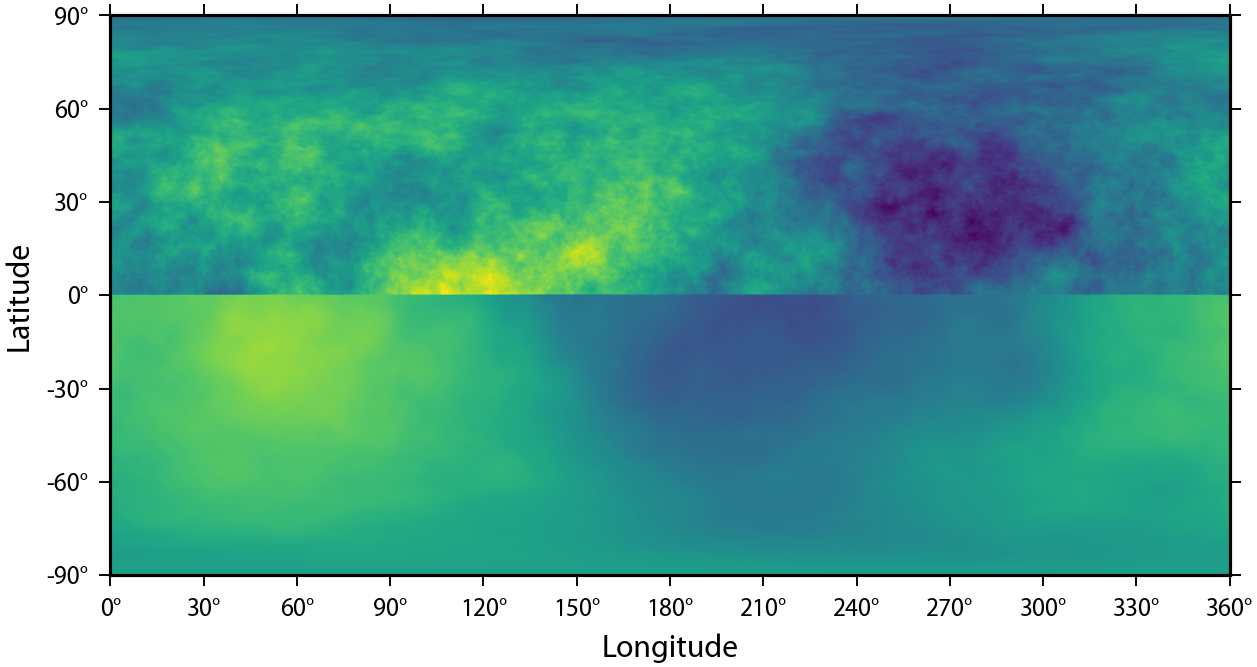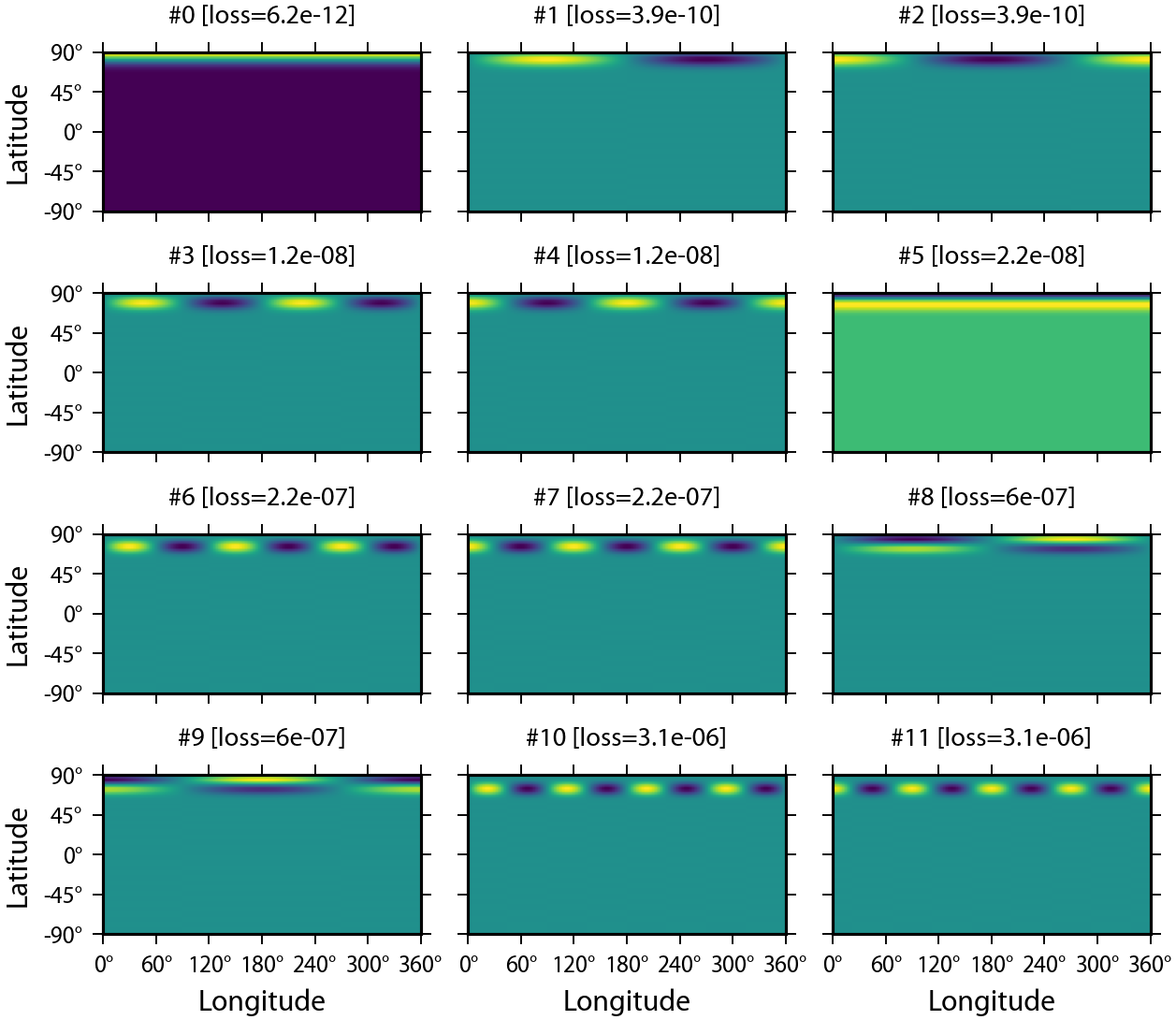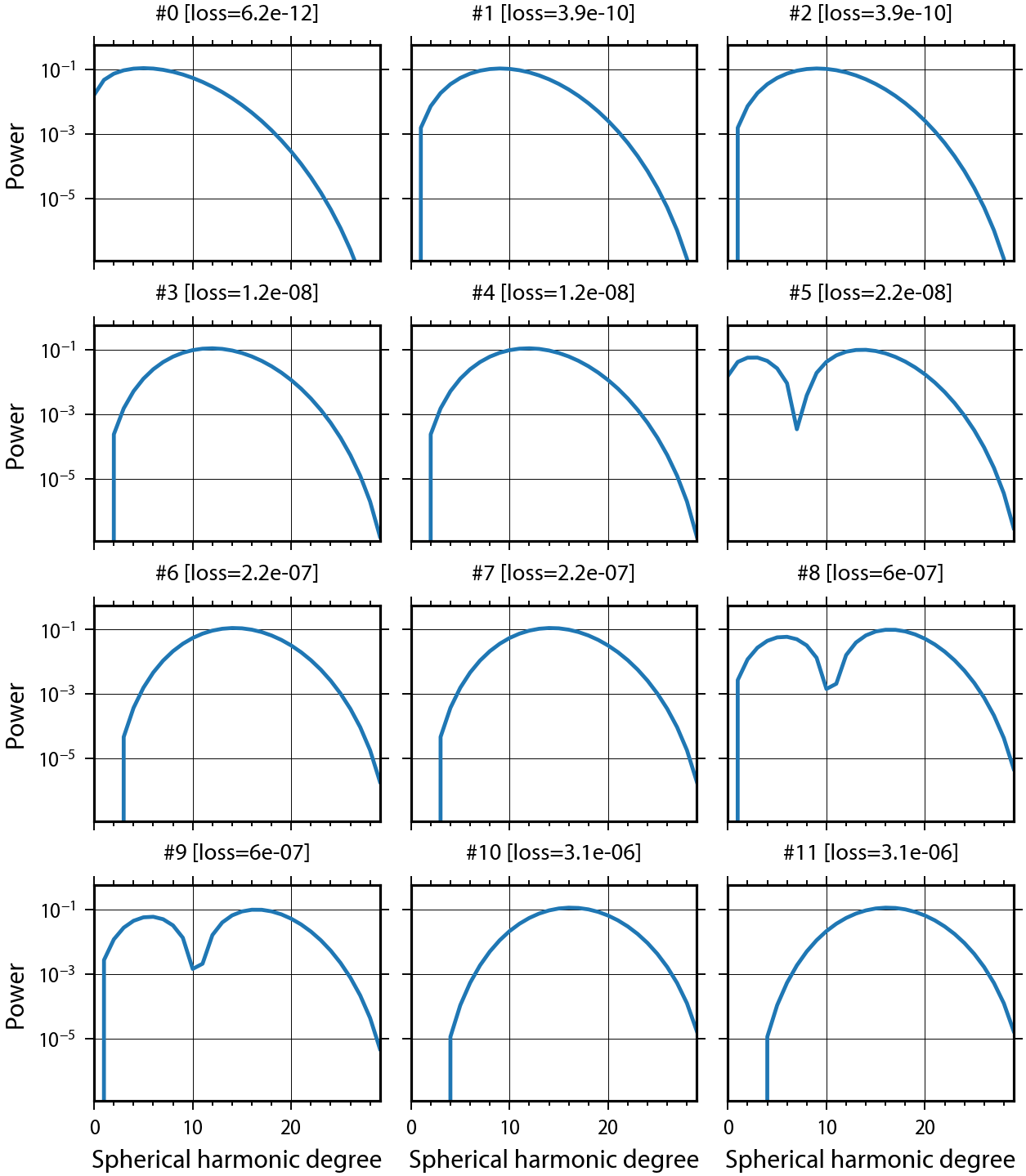# Localization Windows and Spectral Analysis¶

Now that we know how to work with the spherical harmonic coefficient and grid classes, let's move on to the other major feature of pyshtools: localization windows and localized spectral analysis. pyshtools allows for localized spectral analyses using either spherical-cap localization windows or localization windows constructed from an arbitrary mask. The windows are constructed to solve the same optimization problem as the Slepian windows that are used in Cartesian multitaper spectral analysis problems. A single class, SHWindow, bundles the relevant shtools functions. In this notebook, we will demonstrate how to construct optimal localization windows, and how to obtain localized spectral estimates for arbitrary regions on the sphere.

In :
import matplotlib.pyplot as plt
import numpy as np
import pyshtools as pysh

pysh.utils.figstyle(rel_width=0.75)
%config InlineBackend.figure_format = 'retina'  # if you are not using a retina display, comment this line


## Spherical Cap Localization Windows¶

To demonstrate the localized spectral analysis routines, we will make use of a pathological data set. In particular, we will construct a function on the sphere whose power spectrum is different in the northern and southern hemispheres. We first create two grids using random coefficients that have power spectra following power laws with exponents of -2 and -3, up to and including degree 300:

In :
ldata = 300
degrees = np.arange(ldata+1, dtype=float)
degrees = np.inf

power_north = degrees**(-2)
power_south = degrees**(-3)

clm_north = pysh.SHCoeffs.from_random(power_north, seed=12345)
grid_north = clm_north.expand()

clm_south = pysh.SHCoeffs.from_random(power_south, seed=54321)
grid_south = clm_south.expand()


Next, create a new grid that contains data from the northern hemisphere of one grid and the southern hemisphere of the other. The SHGrid method from_array() returns a copy of the data, so you don't need to worry about modifying the original data set:

In :
grid = pysh.SHGrid.from_array(grid_north.data)

nlat = grid_north.nlat
grid.data[nlat//2:nlat+1,:] = grid_south.data[nlat//2:nlat+1,:].copy()
grid.plot(show=False)

clm = grid.expand()Here, we made use of the grid attribute nlat, which is the number of rows of the gridded data, and we modified the raw gridded data for grid that is stored in the data class attribute.

Next, let's construct some spherical-cap localization windows. This is done using the constructor from_cap() that is associated with the SHWindow class. The other option, as we will demonstrate later, is from_mask() and takes as input a user defined mask. For spherical-cap windows, we need to specify both the angular radius of the cap and its spherical harmonic bandwidth, and for this example we will use an angular radius of 30 degrees and a bandwidth of 29.

In :
lwin = 29
capwin = pysh.SHWindow.from_cap(theta=30.,
lwin=lwin)


This command calculates the spherical harmonic coefficients of the spherical cap windows along with their associated angular orders and concentration factors. If you ever need to access these directly, they are stored in the class attributes tapers, orders and eigenvalues. By default, theta should be provided in degrees, but this can be input in radians by specifying the optional parameter theta_degrees=False. If you are curious to see what these windows look like, you can plot them using the built in method plot_windows(). Let's plot the first 12 of them:

In :
fig, axes = capwin.plot_windows(12, loss=True, show=False)As is seen, the windows are centered oven the north pole, 1 minus the concentration factor is noted above the window as the loss factor, and all of these windows are very well localized. To instead provide the concentration factor in the title, just set loss=False. If you want to know how many windows have concentration factors greater than a specified value, you can use the method number_concentrated():

In :
capwin.number_concentrated(0.99)

Out:
34

You can return an SHGrid class instance of an individual window using the method to_shgrid(), you can return the spherical harmonic coefficients of individual windows using to_array() and to_shcoeffs(), and you can also return as a numpy array the power spectra of one or more windows using the method spectra(). If you want to plot the power spectra of the windows, this can be done using the built in method plot_spectra():

In :
fig, axes = capwin.plot_spectra(12, loss=True, show=False)In general, we will not perform localized spectral analyses at the north pole, so let's rotate the windows so that they are centered at 45 degrees north and 90 degrees east. This is easily accomplished using the rotate() method. To speed things up, we will rotate only the first 50 best-localized windows, and not all 900 of them:

In :
capwin.rotate(clat=45.,
clon=90.,
nwinrot=50)

fig, axes = capwin.plot_windows(12, loss=True, show=False)